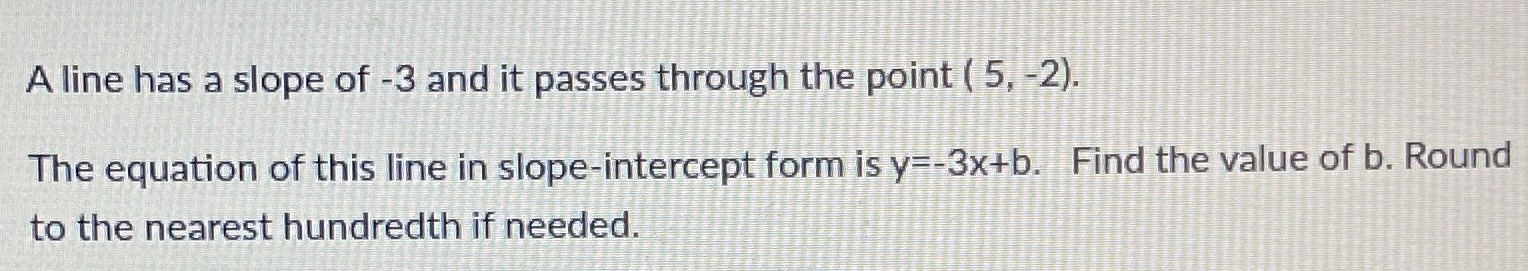### ¿Todavía tienes preguntas de matemáticas?

Pregunte a nuestros tutores expertos
Algebra
PreguntaA line has a slope of $$- 3$$ and it passes through the point $$( 5 , - 2 )$$ . The equation of this line in slope-intercept form is $$y = - 3 x + b$$ . Find the value of b. Round to the nearest hundredth if needed.